Engineering functions trignometric functions of complex numbers imsin, imcos, imtan, imcsc, imsec, imcot

Excel Function IMSIN

The IMSIN() function is used to find the sine of a given complex number.

The IMSIN() function takes a complex number as its argument in the form of x+iy.

The actual formula for Sine of a complex number is as given below: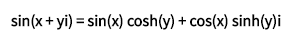Complex Number1 IMSIN Formula 2+5i 67.4789152384559-30.8794313435882i =IMSIN(L4) 4+2.3i -3.81218115678322-3.22701359064241i =IMSIN(L5) 6+5.7i -41.7545593256173+143.48019268019i =IMSIN(L6) 1.4+8i 1468.79228700939+253.332427572756i =IMSIN(L7) 2-8i 1355.28886606391+620.258048292821i =IMSIN(L8)

Excel Function IMCOS

The IMCOS() function is used to determine the cosine value of a given complex number.

This function takes a complex as its parameter and is in the form of x+iy.

The formula for calculating cosine of a complex number is as given below: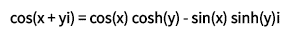Complex Number1 IMCOS Formula 2+5i -30.8822353189167-67.4727884405875i =IMCOS(L17) 4+2.3i -3.29254714427699+3.73630501367712i =IMCOS(L18) 6+5.7i 143.483405376802+41.753624411012i =IMCOS(L19) 1.4+8i 253.33248459038-1468.79195642783i =IMCOS(L20) 2-8i -620.258187894532+1355.2885610286i =IMCOS(L21)

Excel Function IMTAN

The IMTAN() function is used to determine the tangent value of a given complex number.

This function takes a complex as its parameter and is in the form of x+iy.

The formula for calculating tangent of a complex number is as given below: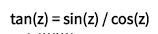Complex Number1 IMTAN Formula 2+5i -0.0000687216388011928+1.000059350149i =IMTAN(L31) 4+2.3i 0.019946061760864+1.00273071774881i =IMTAN(L32) 6+5.7i -0.0000120141609352638+0.999981105397924i =IMTAN(L33) 1.4+8i 7.53959160022853E-08+1.00000021206633i =IMTAN(L34) 2-8i -1.70333827133693E-07-1.00000014711579i =IMTAN(L35)

Excel Function IMCSC

The IMCSC() function returns the cosecant value of a complex number.

The cosecant value is nothing but the inverse of sine value.

This function takes a complex number as its argument.

The formula of cosecant function is as given below: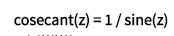Complex Number1 IMCSC Formula 2+5i 0.0122534247739045+0.00560736324365171i =IMCSC(L44) 4+2.3i -0.152815237366451+0.129358188280564i =IMCSC(L45) 6+5.7i -0.00186988547486249-0.00642544269551314i =IMCSC(L46) 1.4+8i 0.000661163046369189-0.000114035211812788i =IMCSC(L47) 2-8i 0.000610070587599244-0.00027920335026741i =IMCSC(L48)

Excel Function IMSEC

The IMSEC() function returns the secant value of a complex number.

The secant value is nothing but the inverse of cosine value.

This function takes a complex number as its argument.

The formula of secant function is as given below: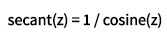Complex Number1 IMSEC Formula 2+5i -0.00560853811692711+0.0122537666692991i =IMSEC(L58) 4+2.3i -0.132759491143768-0.150652346234698i =IMSEC(L59) 6+5.7i 0.00642534375443527-0.00186977294781789i =IMSEC(L60) 1.4+8i 0.000114035285844811+0.000661163177981821i =IMSEC(L61) 2-8i -0.00027920349525829-0.000610070629792488i =IMSEC(L62)

Excel Function IMCOT

The IMCOT() function returns the cotangent value of a complex number.

The cot value is nothing but the inverse of tangent value.

This function takes a complex number as its argument.

The formula of cot function is as given below: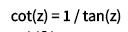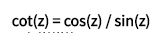<
 Complex Number1 IMCOT Formula 2+5i -0.000068713481923862-0.999940648651408i =IMCOT(L72) 4+2.3i 0.0198297259600569-0.996882270950545i =IMCOT(L73) 6+5.7i -0.0000120146149519776-1.00001889481474i =IMCOT(L74) 1.4+8i 7.53958840244244E-08-0.999999787933708i =IMCOT(L75) 2-8i -1.70333777016106E-07+0.999999852884198i =IMCOT(L76)